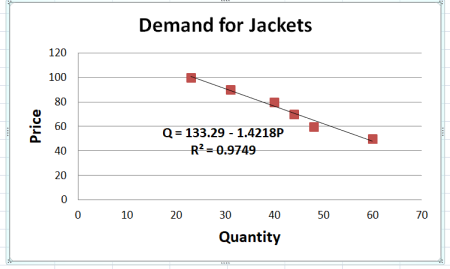# A producer of outdoor clothing used a focus group to obtain information about the demand for...

## Question:

A producer of outdoor clothing used a focus group to obtain information about the demand for fleece jackets with built-in battery operated warming panels. At prices of $100,$90, $80,$70, $60, and$50, the focus group demanded 23, 31, 40, 44, 48, and 60 jackets, respectively.

Use the Excel trend-line option to estimate a linear demand function and to determine the associated Upper R squared R2 statistic? What is the linear demand function?

## Plotting and Fitting a Demand Curve

The trend-line features of Excel can be used to display the relationship between two variables, such as that between price and quantity demanded of a good. The {eq}R^2 {/eq} value and equation specifying the functional relationship between the variables can be generated as well.

The following chart shows the trend-line estimated using Excel.An easy way to produce this is as follows.

• Arrange...

Become a Study.com member to unlock this answer! Create your account